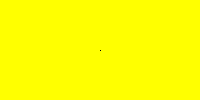# Pixels on an Image

## Pixels

Pixels are a small area of color on a display screen. Images are formed by pixels on the screen. We can manipulate pixels by changing the RGB Value, which are essentially changing the value of red, green and blue color on each pixels. When we see the an image on the screen, it is made of huge numbers of tiny pixel. Each tiny pixels has its only unique position and color it displays.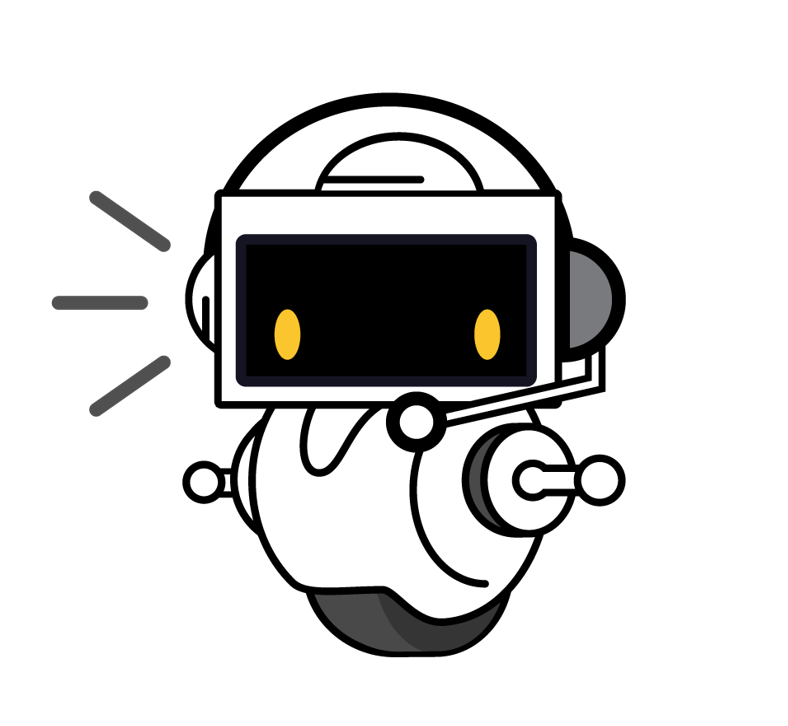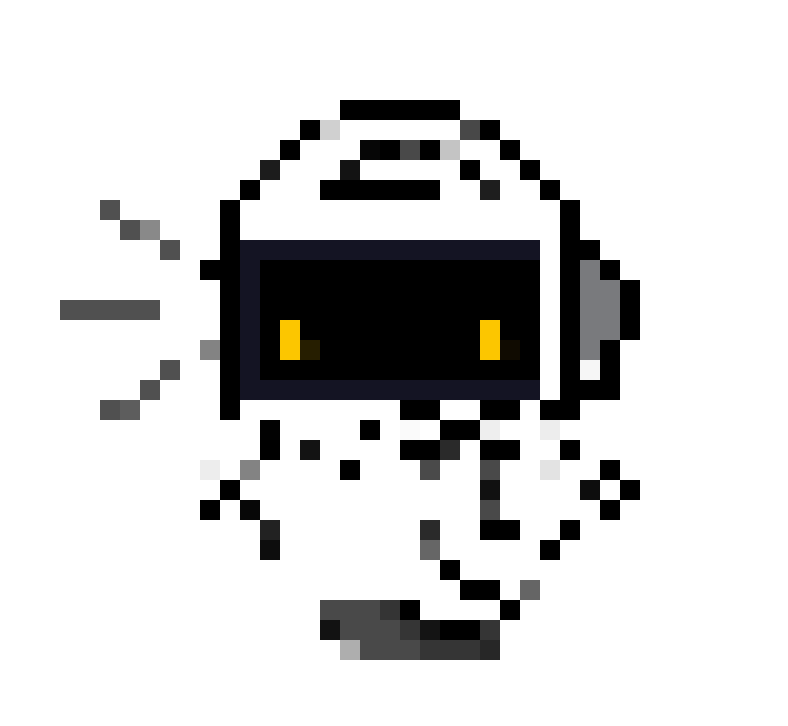Let’s jump into making images. Don’t forget, we learned how to open and save images in Python using the Pillow module in the earlier sections!

## Making images using pixels

``````# Remember to import Image
from PIL import Image

Image.new(mode, size)
Image.new(mode, size, color)
``````

`Image.new()` creates a new image with the given mode and size (and optionally, the color). Here, we can use `RGB` as mode. Size is a `(width, height)` value of an image. Color is the RGB Color of the pixels. We can also use color names rather than RGB values. If you do not initialize color value, the image is filled with black.

## Let’s see some examples

``````# Remember to import Image
from PIL import Image
img = Image.new('RGB', (200,100),(100,100,100))
img.save('pil_grey.png')
``````

The variable `img` stores the PNG image that looks like this: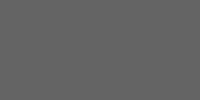``````# Remember to import Image
from PIL import Image
img=Image.new('RGB', (200,100),"black")
img.save('pil_black.png')
``````

Here, specifying `black` as the RGB color creates and stores the PNG image that looks like this: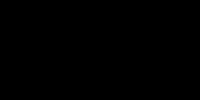## Changing a pixel in an image

What if we want to put another pixel in an image?

The function `img.putpixel( (x,y), (r, g, b))` puts a new pixel on the image with the given position and color. Position is a `(width, height)` value of that pixel’s location on the image. Color is the RGB Color of that pixel.

## Let’s see an example

``````# Remember to import Image
from PIL import Image
img=Image.new('RGB', (200,100),"yellow")
img.putpixel( (100,50), (0, 0, 0))
img.save('pil_black-dot.png')
``````

After creating a 200x100 yellow image, the `putpixel` function puts a tiny small dot in the middle of this yellow block. This is one small pixel - in fact, it’s so small you may have to expand the image to actually see it!